# Quantitative trading on cryptocurrency market Q3

This is the second chapter of a learning process that started last September.

# Third Quarter

The third step is defined for the next 3 months, where the main goal is to define a specific strategy of quantitative trading and work on it with real money on crypto currency market.

Following the V2MOM model:

• Vision: Have a strategy running in crypto currency market running not with a period of 2 – 3 hours, but some days (stop operating at 3m).
• Values: have fun, learn a lot, build a team with Dani, do practices and more practices.
• Method: learn about trading basis, do backtesting with Quantopian on stocks or Forex (analyze the results in deep).
• Obstacles: Time.
• Measures:
• Make short/long decisions based on 1 hour.
• Perform backtesting with Quantopian and document the results and findings.
• Improve and document the “mode operations” and “mode backtesting”.

Death line = June 2018

# Results (July 1st, 2018)

• Time to be accountable, let’s go…
• I have done more than 50 operations, being May and June with negative results. July has been with 34 operations and positive global results.
• I have learned to trade with a bearish market, and interestingly I have issues to work with a bullish market (I short too soon).
• I have not worked with Quantopian nor tradingview on any backtesting, this was a bid fault.
• I discovered an interesting indicator: VPCI, Volume price confirmation indicator. It really helps to indentify real moves of the market.
• I have been able to cultivate patience during these months, but some days I did some moves that did not make sense. I have to evolve on this.
• I finish read one of the fundamental analysis books.
• I have started to apply the knowledge I’m acquiring on the medium term market I work on stocks.
• Not too much.
• I need to retake the back testing exercises and continuing the practices.

# The basis

• Support vector machine (SVM) is a supervised learning method.
• It can be used for regression or classification purposes.
• SVM is useful for hyper-text categorization, classification of images, recognition of characters…
• The basic visual idea is the creation of planes (lines) to separate features.
• The position of these planes can be adjusted to maximized the margins of the separation of features.
• How we determine which plane is the best? well, it’s done using support vectors.

# Support vectors

The support vectors are the dots that determine the planes. The orange and blue dots generate lines and in the middle you can find what is called the hyper-plane.

The minimum distance between the lines created by the support vectors is called the margin.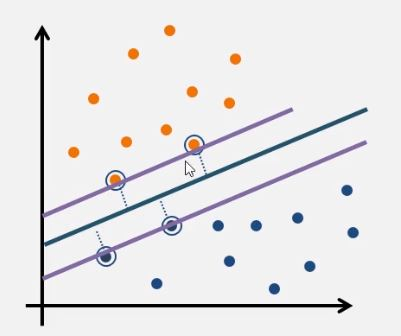The diagram above represents the more simple support vector draw you can find, from here you can make it more complex

# Before to start

What is an error?

Observation prediction error = Target – Prediction = Bias + Variance + Noise

# The main sources of errors are

• Bias and Variability (variance).
• Underfitting or overfitting.
• Underclustering or overclustering.
• Improper validation (after the training). It could be that comes from the wrong validation set. It is important to divide completely the training and validation processes to minimize this error, and document assumptions in detail.

#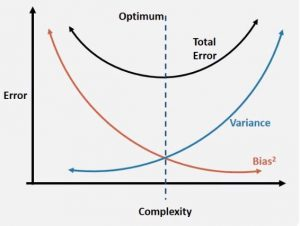Underfitting

This phenomenon happens when we have low variance and high bias.

This happens typically when we have too few features and the final model we have is too simple.

How can I prevent underfitting?

• Increase the number of features and hence the model complexity.
• If you are using a PCA, it applies a dimension reduction, so the step should be to unapply this dimension reduction.
• Perform cross-validation.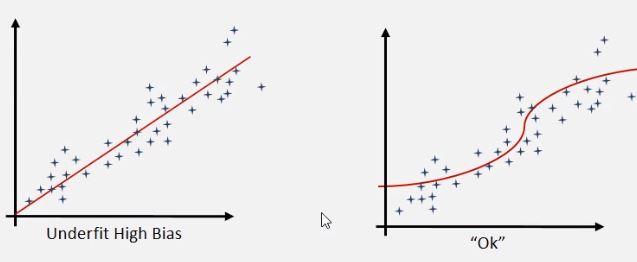# Overfitting

This phenomenon happens when we have high variance and low bias.

This happens typically when we have too many features and the final model we have is too complex.

How can I prevent overfitting?

• Decrease the number of features and hence the complexity of the model.
• Perform a dimension reduction (PCA)
• Perform cross-validation.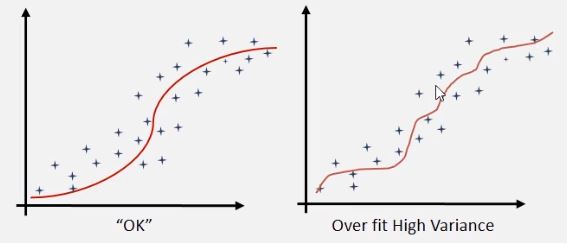# Cross validation

This is one of the typical methods to reduce the appareance of errors on a machine learning solution. It consist on testing the model in many different contexts.

You have to be careful when re-testing model on the same training/test sets, the reason? this often leads you to underfitting or overfitting errors.

The cross-validation tries to mitigate these behaviors.

The typical way to enable cross validation is to divide the data set in different sections, so you use 1 for testing, and the others for validations. For instance, you can take a stock data set from 2010 to 2017, use the data from 2012 as testing dataset and use the other divisions by year for validation of your trading model.

# Neural networks

They can be used to avoid that errors are backpropagated. The neural network helps you to minimize the error by adjusting the impact of the accumulation of data.

# The basis

• K-means clustering is an unsupervised learning method.
• The aim is to find clusters and the CentroIDs that can potentially identify the
• What is a cluster? a set of data points grouped in the same category.
• What is a CentroID? center or average of a given cluster.
• What is “k”? the number of CentroIDs

# Typical questions you will face

• The number k is not always known upfront.
• First look for the number of CentroIDs, then find the k-values (separate the 2 problems).
• Is our data “clusterable” or it is homogeneous?
• How can I determine if the dataset can be clustered?
• Can we measure its clustering tendency?

#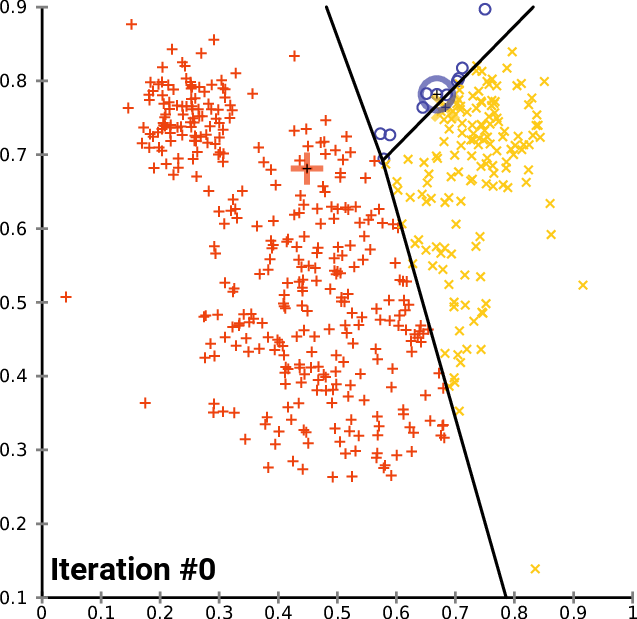A real situation: identification of geographical clusters

You can create a K-means algorithm, where the distance is used to know the similarities or dissimilarities. Any pointers how a properties of observations are mapped so that you can decide the groups based on the K-means or hierarchical clustering.

Since k-means tries to group based solely on euclidean distance between objects you will get back clusters of locations that are close to each other.

To find the optimal number of clusters you can try making an ‘elbow’ plot of the within group sum of square distance.

http://nbviewer.jupyter.org/github/nborwankar/LearnDataScience/blob/master/notebooks/D3.%20K-Means%20Clustering%20Analysis.ipynb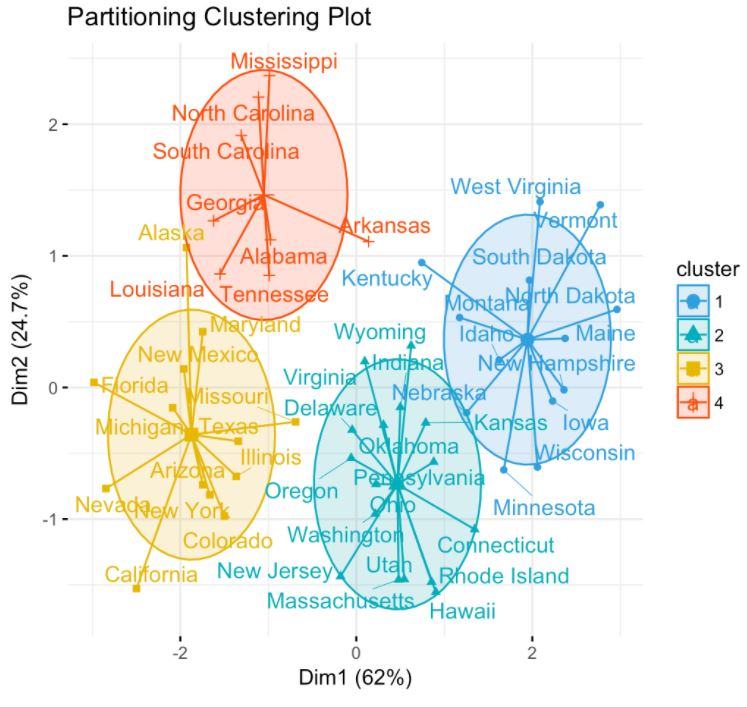.

# The basis

• It’s based on Bayes’ theorem (check the wikipedia link, and see how complex the decision trees could be).
• Assumes predictors contribute independently to the classification.
• Works well in supervised learning problems.
• Works with continuous and discrete data.
• Can work with discrete data sets.
• It is not sensitive to non-correlating “predictors”.

# Example: spam/ham classification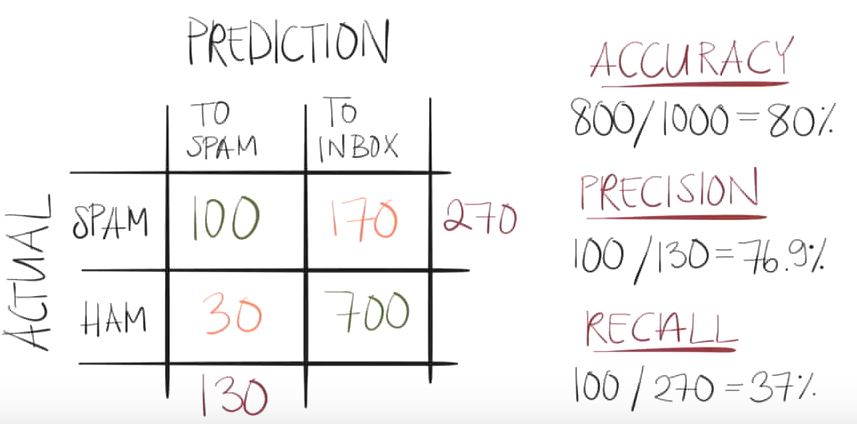# The basis

• Logistics or Logit regression.
• It’s a regression model where the dependent variable (DV) is categorical.
• Outcome follows a Bernoulli distribution.
• Success = 1 , failure = 0.
• Natural logarithm of odds ratio ln (p/1-p)… logit(p).
• Inverse log curve gives a nice “s” curve to work with.
• Equate logarithm of odds ratio with regression line equation.
• Solve for probability p

# Example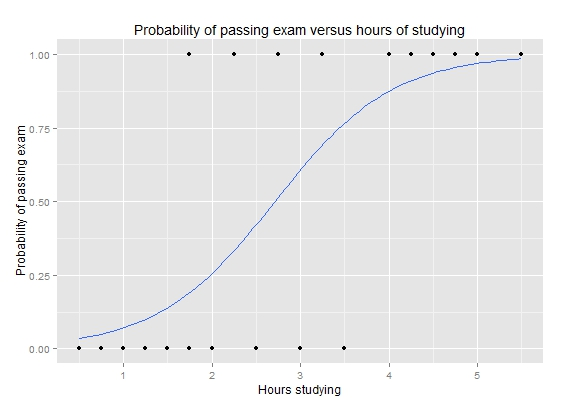Continue learning the basis !

# Machine Learning as a Service

I have learned a lot about pros and cons of each solution reading the article.

This table summarizes the current situation of each product: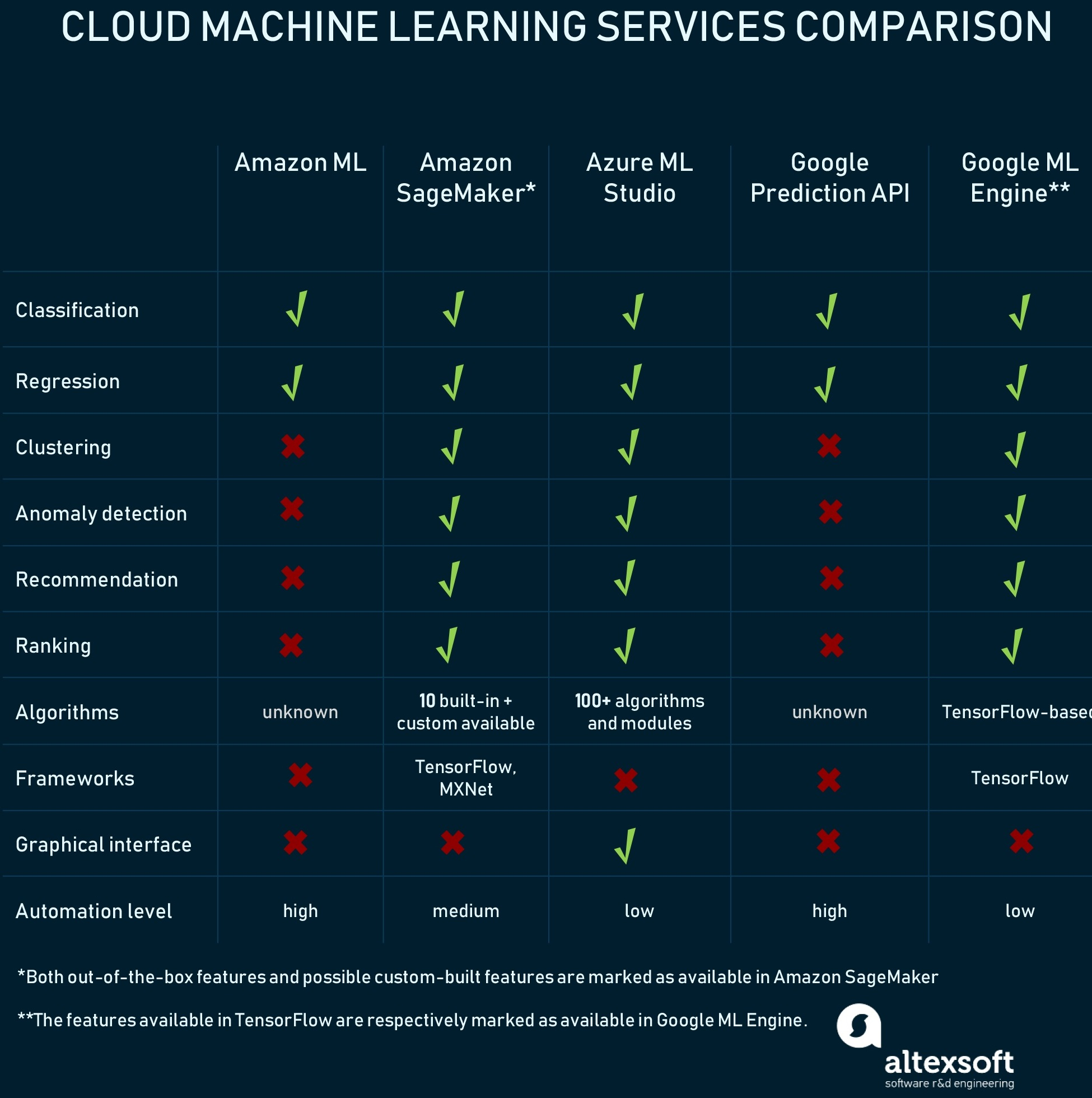# What is overfitting?

when you are preparing a machine learning solution, you work basically with data sets that contains:

• Data: relevant and/or important data.
• Noise: inrelevant and/or non important data.

With this data you want to identify a trigger, a signal that responds to your target pattern you want that your code identifies.

So you start identifying a pattern and you work to improve it.

Suddenly, you improve your pattern identification so much, till a point where you will be not just using the data but your pattern is also using the noise side of the data to trigger the signal.

This phenomenon is not desired, and it is what is called overfitting.

In the picture from the left:

• The black line represents a healthy pattern.
• The green line represents an overfitted pattern.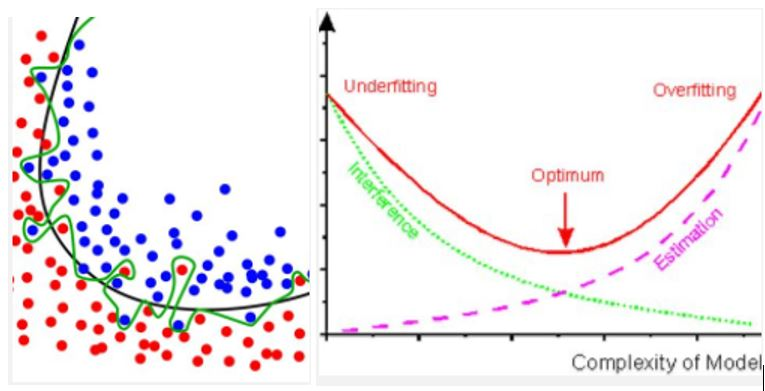# Understanding machine learning

I have watched this video from @TessFerrandez: Machine Learning for Developers.

The video explains how the process of building a machine learning solution is. She explains it in plain English and with very nice examples easy to remind the concepts.

The video helped me to link a lot of technical ideas explained in the courses with a natural flow. Now all make sense to me.

# When do I need a machine learning solution?

Imagine that you have this catalogue of pictures: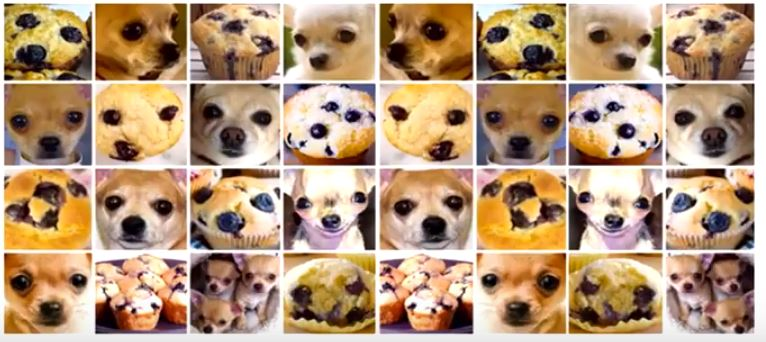and that you want to identify when a picture has a muffin or a chihuahua.

The traditional way to do it is using “if / else” sentences, like for example: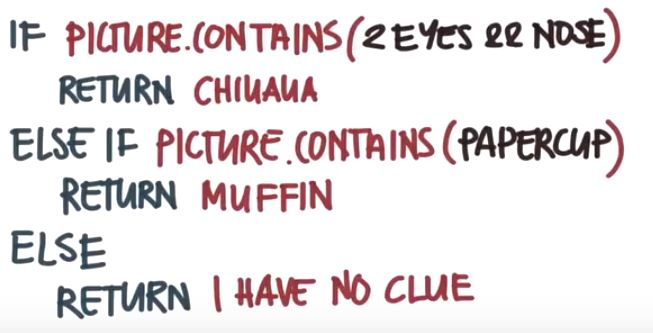The results are not going to be good, why?

• The problem is more complex than the basic questions you are doing and it requires thounsand of combinations of conditional sentences.
• To find the right sequence of conditional sentences can take years.

Here, is when machine learning techniques can help you. At the end of the day, it’s a different approach to find a solution to a complex problem.

# What are the steps to perform a machine learning solution?

The basic steps to build a machine learning solution are:## 1.- Ask a sharp question: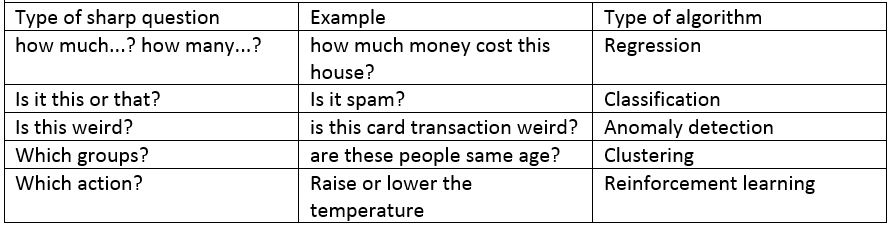At the end of the day, depending on the question you ask, you will use a different machine learning techniques.

What type of machine learning technique can I use? Well there are so many of them, but these are the basic ones:

• Supervised learning : learning model based on a set of labeled examples. For instance you want to identify when there is a cat in a picture and you use a set of pictures where you know that there are indeed cats.
• Unsupervised learning: think about the a data set of population where you use a clustering algorithm to classify the people in five different groups, but we do not say in which type of groups. For instance when we are looking for movies recommendations and suddenly there is a pattern identified by age (which initially we did not know it was a cluster of relevant data that we could cluster or classify)
• Reinforcement : it uses the feedback to make decisions. For instance, a system that measures the temperature, then it compares with the target temperature and finally raise or lower the temperature. This reminds me to the servo-systems and fuzzy logic used at electronic level when I studied electronic engineering.

## 2.- Collect data

look for databanks, there are so many on the internet. For sure if you want precise trading data from a good bunch of markets and thousands of parameters, you will have to pay for it.

## 3.- Explore data: relevant, important and simple.

• Relevant: determine features, define relevant features, discard irrelevant features.
• Important: define important data.
• Simple: it has to be simple (for instance avoid GPS coordinates, and replace by distance to a lake).

## 4.- Clean data:

• Identify duplicate observations,
• complete or discard missing data,
• identify outliers,
• identify and remove structural errors.

This step is a tedious process, but it also helps to understand the data.

## 5.- Transform features

Do things like to turn the GPS coordinates into distance to a lake,

## 6.- Select algorithms

the base algorithms are:

• Linear regression,
• decision tree,
• naive bias
• logistics regression
• neural nets: basically is the combination of different layers of data and algorithms.

The more complex algorithms are composed in so many cases by the base algorithms. It could be neural nets or if we make it more complex we will build neural architectures.

As it is reflected on the table above, the election of the algorithm depends on the question we want to answer.

## 7.- Train the model

Apply the algorithms to the cleaned datasets and do a fine tune of the algorithm.

## 8.- Score the model

Test the model and evaluate how good/bad it is.

Typical metrics are:

• Accuracy: how many of the total ones were classified correctly?
• Precision: how many decisions were done correctly?
• Recall: how many of the specific decision were correct?